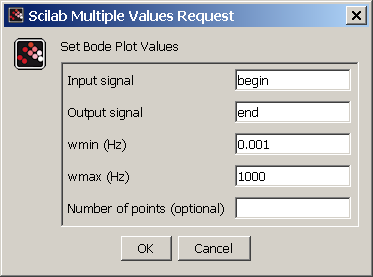# Control and Power Systems module

Although Scilab includes many functions for plotting various characteristics of linear control systems (e.g. step response), these functions cannot be easily applied to Xcos diagrams.

The Equalis Control and Power Systems Toolbox  offers a new palette of Xcos blocks which allows the user to view plots of important characteristics a control system defined in Xcos diagram.

These characteristics include Step Response, Bode and Nyquist plots (optionally which gain and phase margins), Nichols plot, Pole-Zero plot, and Singular values plot.In this example we sill use Control and Power Systems Toolbox to analyze a linear model of a permanent magnet DC motor. This motor can be modeled by an electrical circuit in the armature as shown below.

Assuming a constant load, we can consider a DC motor as a SISO sysem, where the applied voltage Va is the input and the motor speed is the output. Using standard Xcos blocks (summation, gain, integration), let us create an diagram of this SISO system.

The resulting diagram is shown below:The input Va can be connected to a constant block (this means a constant voltage).

The output w is the speed in rad/s.

Let us use Simulation-]Set context in Xcos to set the following values of constants which appear in the diagram:

J=3.2284E-6; b=3.5077E-6; K=0.0274; R=4; L=2.75E-6;

Now let us analyze the obtained SISO system using Control and Power Systems Toolbox. For example, let us build a Bode plot.

To do this, we open the Linear Analysis palette and put two Operating_Point blocks and one Bode block on the diagram.

The Operating_Point blocks indicate inputs and outputs of a linear system or subsystem in a diagram.

They have no effect on simulation.

The only parameter an Operating_Point block is its name (the names of different blocks should be unique).

Let us set the names of two Operating_Point blocks as "begin" and "end" and connect them as displayed follows:The role of Bode block is to build a bode plot of a SISO system located between two operating points ("begin" and "end").

Let us set its parameters as follows:When at least one Bode block is present in the diagram and the user clicks Simulation-]Start, Xcos does not start a simulation, but instead builds and displays a Bode plot.

Note that to run a normal simulation the user can (temporally) remove Bode block (and all other Linear Analysis blocks except Operating_Point).

Now let us start simulation. Xcos will show the Bode plot of the transfer function of our system.Analogously, we can build a Nyquist plot and all other plots supported by Control and Power Systems Toolbox.

 http://www.equalis.com/